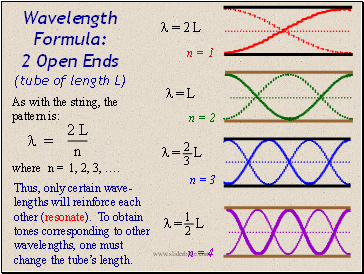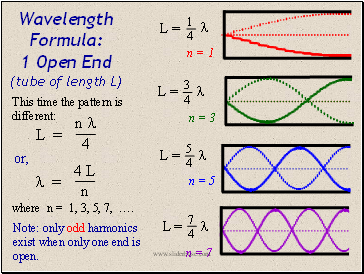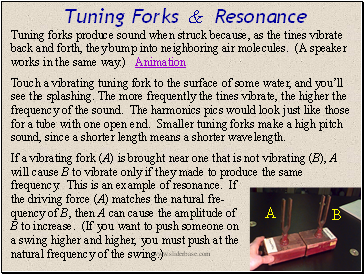# SoundPage 9

#### WATCH ALL SLIDES

n = 1 (fundamental)

Harmonics animation

1st, 2nd, and 3rd Harmonics

Slide 35Wavelength Formula: 2 Open Ends (tube of length L)

As with the string, the pattern is:

Thus, only certain wave-lengths will reinforce each other (resonate). To obtain tones corresponding to other wavelengths, one must change the tube’s length.

where n = 1, 2, 3, ….

n = 2

 = L

n = 1

 = 2 L

 = L

1 2

n = 4

n = 3

 = L

2 3

Slide 36Standings Waves in a Tube: 1 Open End

Harmonics animation

1st, 3rd, and 5th Harmonics animations (scroll down)

n = 1 (fundamental)

If a tube has one open and one closed end, the open end is a region of maximum vibration of air molecules—an antinode. The closed end is where no vibration occurs—a node. At the closed end, only a small amount of the sound energy will be transmitted; most will be reflected. At the open end, of course, much more sound energy is transmitted, but a little is reflected. Only certain wavelengths of sound will resonate in this tube, which depends on it length.

Slide 37Wavelength Formula: 1 Open End (tube of length L)

This time the pattern is different:

Note: only odd harmonics exist when only one end is open.

where n = 1, 3, 5, 7, ….

n = 3

n = 1

L =

n = 7

n = 5

1 4

L = 

5 4

L = 

7 4

or,

Slide 38## Tuning Forks & Resonance

Tuning forks produce sound when struck because, as the tines vibrate back and forth, they bump into neighboring air molecules. (A speaker works in the same way.) Animation

Touch a vibrating tuning fork to the surface of some water, and you’ll see the splashing. The more frequently the tines vibrate, the higher the frequency of the sound. The harmonics pics would look just like those for a tube with one open end. Smaller tuning forks make a high pitch sound, since a shorter length means a shorter wavelength.

If a vibrating fork (A) is brought near one that is not vibrating (B), A will cause B to vibrate only if they made to produce the same frequency. This is an example of resonance. If the driving force (A) matches the natural fre- quency of B, then A can cause the amplitude of B to increase. (If you want to push someone on a swing higher and higher, you must push at the natural frequency of the swing.)

A

B

Slide 39## Resonance: Shattering a Glass

determined by flicking the glass with your finger and listening to the tone it makes. If the glass is being bombarded by sound waves of this freq-uency, the amplitude of the vibrating glass with grow and grow until the glass shatters.

Go to page:
1  2  3  4  5  6  7  8  9  10  11  12  13